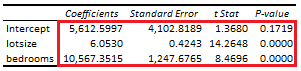# Linear Regression: Coefficients Analysis

Last Update: February 21, 2022

Linear Regression: Coefficients Analysis is used to analyze linear relationship between one dependent variable  and two or more independent variables . Variable  is also known as target or response feature and variables  are also known as predictor features. It is also used to evaluate whether adding independent variables individually improved linear regression model.

As example, we can fit a three-variable multiple linear regression with formula . Regression fitted values  are the estimated  values. Estimated constant coefficient  is the  value when  and . Estimated partial regression coefficient  is the estimated change in  when  changes in one unit while holding  constant. Similarly, estimated partial regression coefficient  is the estimated change in  when  changes in one unit while holding  constant.

Then, we can estimate coefficient  standard error with formula  as squared root of residual mean squared error  multiplied by  element of matrix  principal diagonal.

Residual mean squared error with formula  is estimated as residual sum of squares  divided by residual degrees of freedom . Residual sum of squares  with formula  is estimated as the sum of squared regression residuals . Regression residuals  with formula  are estimated as differences between actual  and fitted  values. Residual degrees of freedom  with formula  are the number of observations  minus number of independent variables  minus constant term.

Matrix  with dimension  x  is the inverse of the matrix product between the transpose of matrix  and matrix . Matrix  with dimension  x  is the independent variables matrix including constant term column of ones.

Next, we can estimate coefficient  t-statistic with formula  and do t-test with individual null hypothesis that independent variable  coefficient is equal to zero with formula . If individual null hypothesis is rejected, then adding independent variable  improved linear regression model.

Below, we find an example of coefficients analysis from multiple linear regression of house price explained by its lot size and number of bedrooms .Table 1. Microsoft Excel® coefficients analysis from multiple linear regression of house price explained by its lot size and number of bedrooms.

Courses

My online courses are hosted at Teachable website.

For more details on this concept, you can view my Linear Regression Courses.

 Data Description: Sales prices of houses sold in the city of Windsor, Canada, during July, August and September, 1987.

Original Source: Anglin, P., and Gencay, R. (1996). Semiparametric Estimation of a Hedonic Price Function. Journal of Applied Econometrics, 11, 633–648.

Source: AER R Package HousePrices Object. Christian Kleiber and Achim Zeileis. (2008). Applied Econometrics with R. Springer-Verlag, New York.

+